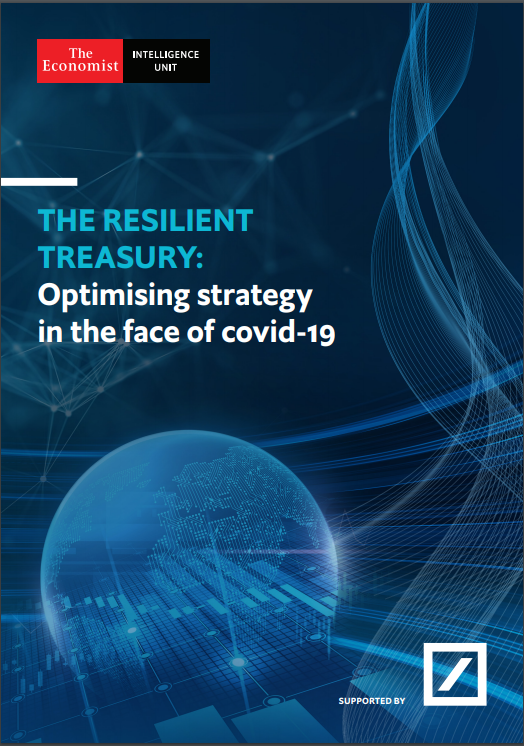Array (  => 0  => 0  => 0  => 0  => 0  => 0  => 0  => 0  => 0  => 0  => 0  => 2  => 0  => 0  => 0  => 0  => 0  => 0  => 0  => 0  => 0  => 0  => 0  => 0  => 0  => 0  => 0  => 0  => 0  => 0  => 0  => 0  => 0  => 0  => 0  => 0  => 0  => 0  => 0  => 0  => 0  => 0  => 0  => 0  => 0  => 0  => 0  => 0  => 0  => 0  => 0  => 0  => 0  => 0  => 0  => 0  => 0  => 0  => 0  => 0  => 0  => 0  => 0  => 0  => 0  => 0  => 0  => 0  => 0  => 0  => 0  => 0  => 0  => 0  => 0  => 0  => 0  => 0  => 0  => 0  => 0  => 0  => 0  => 0  => 0  => 0  => 0  => 0  => 0  => 0 )

## The resilient treasury: In conversation with Ole MatthiessenDownload the report from The Economist Intelligence Unit, supported by Deutsche Bank, to explore the priorities that treasurers will pursue up to 2025.

A new report written by The Economist Intelligence Unit (EIU) “The resilient treasury: Optimising strategy in the face of covid-19” explores the forces that will shape and define the corporate treasury function and the priorities over the coming decade. It identifies the macro and financial risks that impact strategy as drivers of strategic change, the regulatory initiatives that are currently top of mind and the technologies and the skills that the function requires. The research, supported by Deutsche Bank, is based on a global survey of 300 corporate treasury executives (conducted between April and May 2020).

Plus watch Ole Matthiessen, Global Head of Cash Management, as he joins EuroFinance to discuss the key findings of the report, and share his views on the relationship between banks and treasury.